# 4. Find the equation of the tangent line to the implicit curve x? - y +...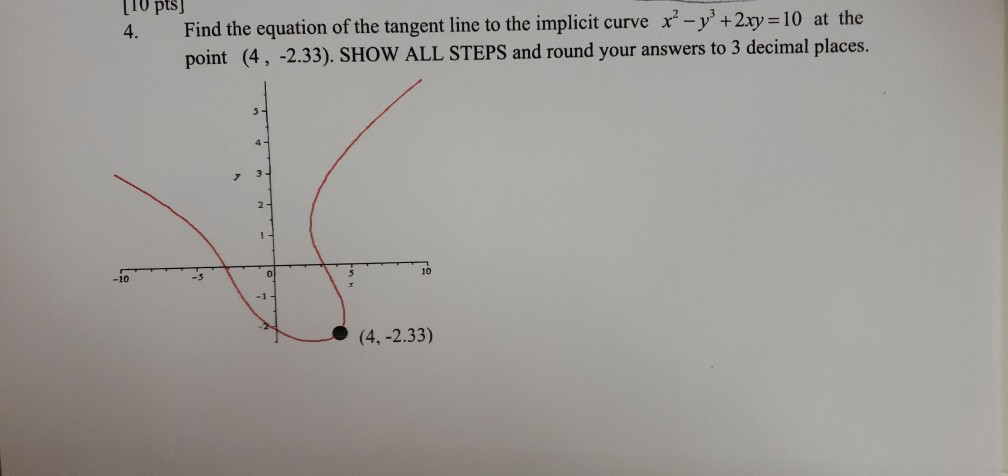4. Find the equation of the tangent line to the implicit curve x? - y + 2xy = 10 at the point (4, -2.33). SHOW ALL STEPS and round your answers to 3 decimal places. 5 - 4- 3 2 1 10 -10 -5 O (4, -2.33)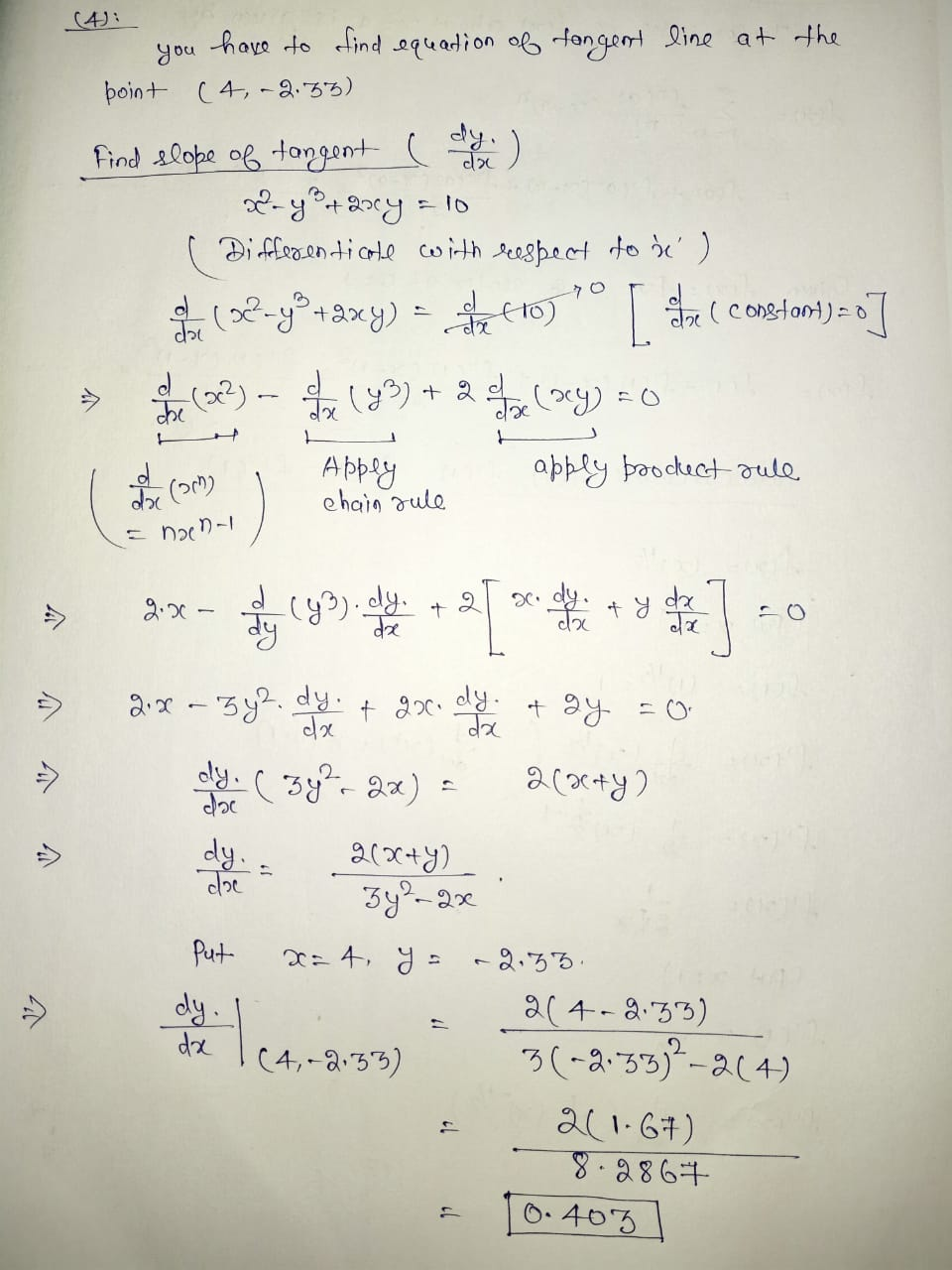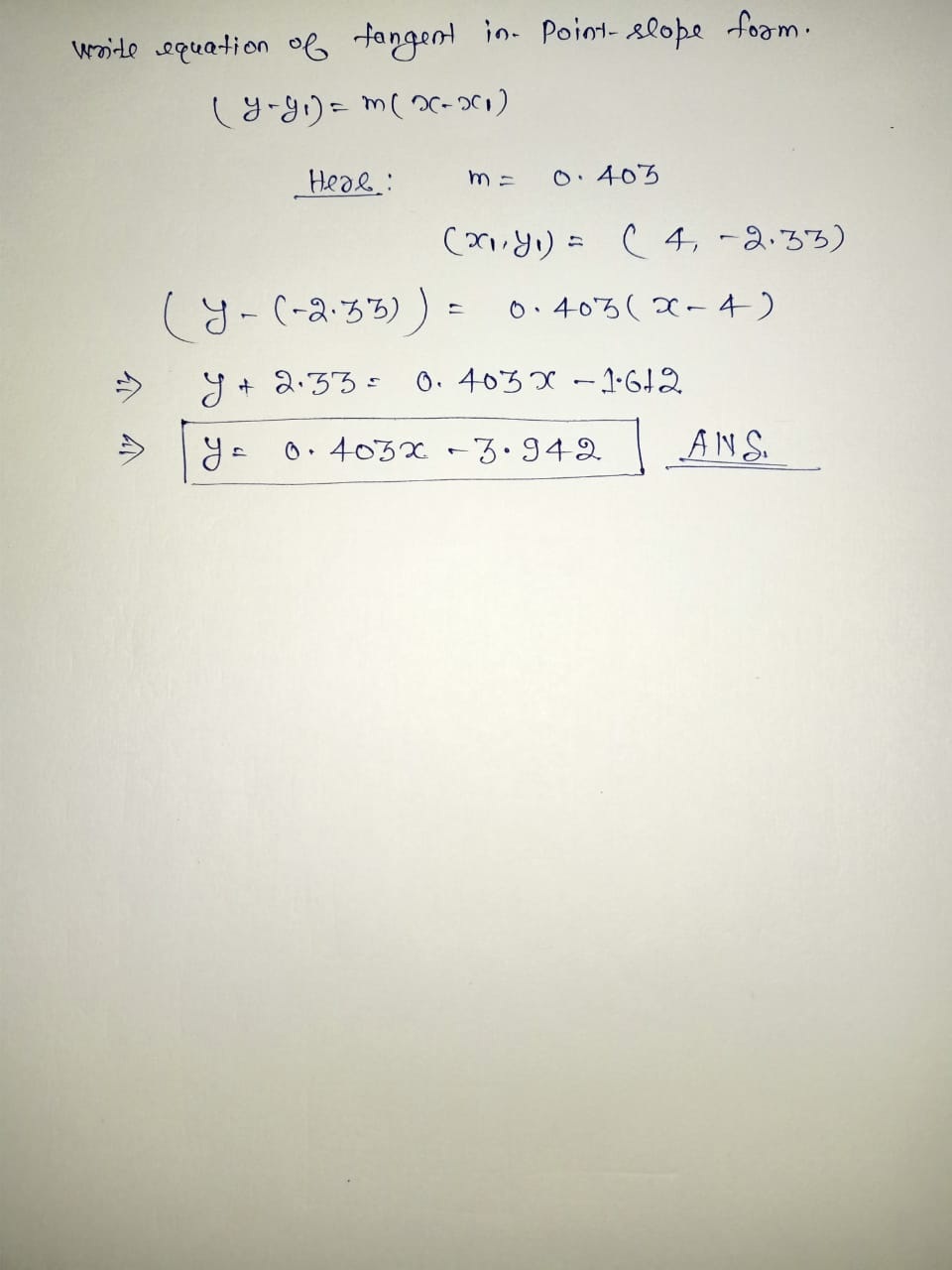#### Earn Coin

Coins can be redeemed for fabulous gifts.

Similar Homework Help Questions
• ### Use implicit differentiation to find the equation of the tangent line to the curve xy^3+2xy=9 at the point (31)

Use implicit differentiation to find the equation of the tangent line to the curve xy^3+2xy=9 at the point (31). The equation of this tangent line can be written in the form y=mx+b where m is

• ### (1 point) Use implicit differentiation to find an equation of the tangent line to the curve...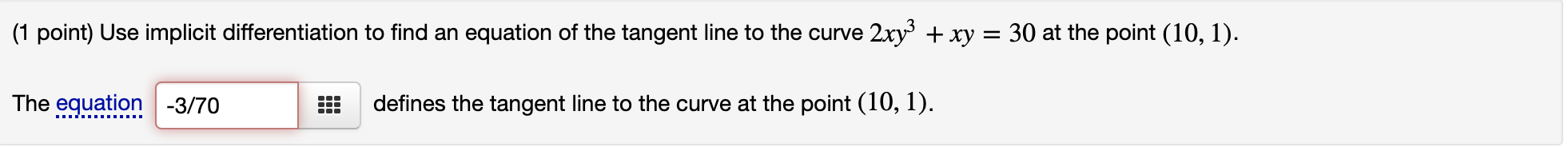(1 point) Use implicit differentiation to find an equation of the tangent line to the curve 2xy3+xy=302xy3+xy=30 at the point (10,1)(10,1). (1 point) Use implicit differentiation to find an equation of the tangent line to the curve 2xy3 + xy = 30 at the point (10, 1). The equation -3/70 defines the tangent line to the curve at the point (10, 1).

• ### Find the equation of the tangent line to the curve at the given point using implicit...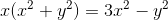Find the equation of the tangent line to the curve at the given point using implicit differentiation. Remember: equation of a line can be found by y-y1=m(x-x1) where m is the slope of the line and (x1,y1) is any point on the line. Curve: at (1,1)

• ### Use implicit differentiation to find the equation of the tangent line to the curve xy^3+xy=5 at the point (4,1)

Use implicit differentiation to find the equation of the tangent line to the curve xy^3+xy=5 at the point (4,1) . The equation of this tangent line can be written in the form y = mx+b where m is:? and where b is:?

• ### Find an equation for the line tangent to the curve at the point defined by the...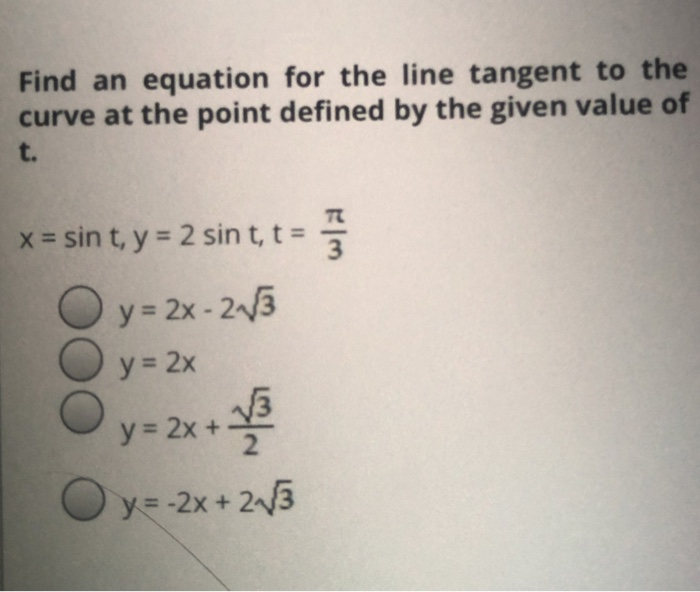Find an equation for the line tangent to the curve at the point defined by the given value of t. x = sin t, y = 2 sin t, t = wa y = 2x - 213 y = 2x y = 2x + 13 Oy=-2x+ 2/3 Find an equation for the line tangent to the curve at the point defined by the given value of t. x=t, y= V2t, t = 18 y=- X-3 y=+x+3 O y = 1...

• ### Use implicit differentiation to find the equation of the tangent line to the curve xy3+xy=14 at the point (7,1)

Use implicit differentiation to find the equation of the tangent line to the curve xy3+xy=14 at the point (7,1) . The equation of this tangent line can be written in the form y=mx+bi dont seem to no how to find m or b

• ### use implicit differentiation to find the slope of the tangent line to the curve (y)/(x-7y)=x^6+5 at the point (1,6/43)

use implicit differentiation to find the slope of the tangent line to the curve (y)/(x-7y)=x^6+5 at the point (1,6/43)

• ### Find an equation of the tangent line to the curve at the given point. y =...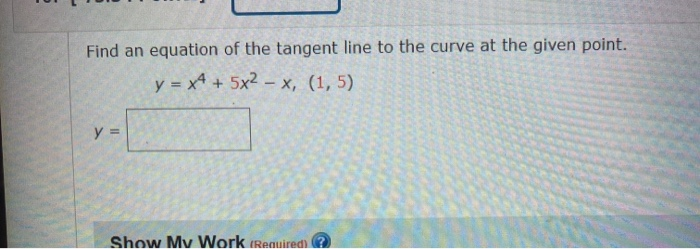Find an equation of the tangent line to the curve at the given point. y = x4 + 5x2 - x, (1,5) y = Show My Work (Requiredi 2

• ### Use implicit differentiation to find the slope of the tangent line to the curve 5x^3 y^2...

Use implicit differentiation to find the slope of the tangent line to the curve 5x^3 y^2 - 4x^2 y = 1at the point (1,1) m=

• ### 13) Find an equation of the tangent line to the curve y=sin(5x)+cos(8x) at the point (π/6,y(π/6)). what is the tangent l...

13) Find an equation of the tangent line to the curve y=sin(5x)+cos(8x) at the point (π/6,y(π/6)). what is the tangent line: 14) f(x)=4x^2cos(4x) what is the first and second derivatives and solve both for F(5) NOTE There should be four answers! 16) Suppose that f(x)=3x/(4−5x^)3 find an equation for the tangent line to the graph of f at x=2. the tangent line: y=